# Size of Atoms and Ions

Remarks:
The distance between the two nuclei is called the "Bond Distance".
If the two atoms which make up the molecule are the same, then half the bond distance is called the "Covalent Radius" of the atom.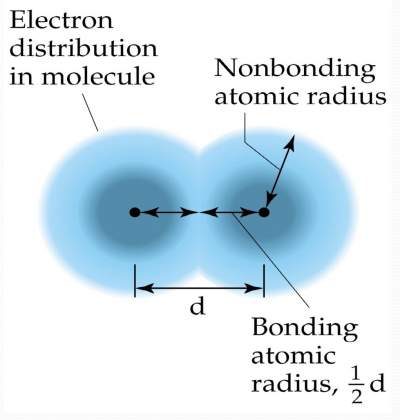The size of the s orbitals in each atom:
3s > 2s > 1s
Similarly, 3p > 2p and 5d > 4d > 3d
Hence, as the principal quantum number (n) increases, the size of the orbital increases.So the question is: How does the trend of atom size look like in a periodic table?

## Atoms become smaller as we move across a period

 As we move across the periodic table, the number of core electrons remains constant, while the nuclear charge increases. Hence, there is an increased attraction between the nucleus and the outermost electrons. This attraction causes the atomic radius to decrease. (The rule of Zeff)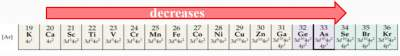## Atoms become larger as we move down a group

 As the principle quantum number (n) increases (by moving down a group), the distance of the outermost electron from the nucleus becomes larger, and the atomic radius increases.(The rule of n)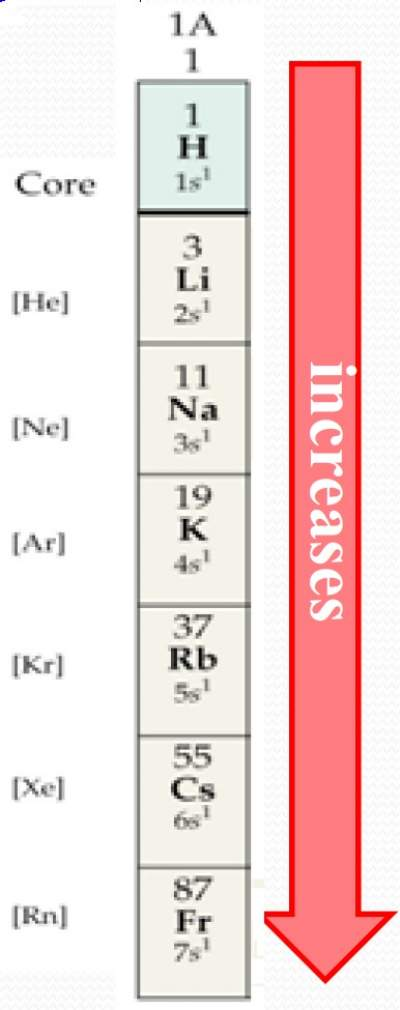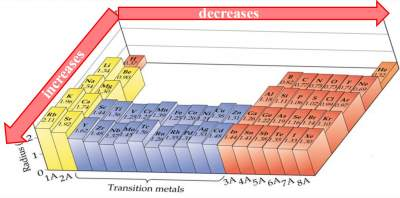So, the question is: How does the trend of ion sizelook like in a periodic table?
Ion size also depends on:
1. Nuclear charge
2. Number of electrons3
3. Orbitals that contain the valence electrons

### Exercise on Comparing Sizes of Atoms and Ions

Cations vacate the most spatially extended orbital and are smaller than the parent atom.
Anions add electrons to the most spatially extended orbital and are larger than the parent atom.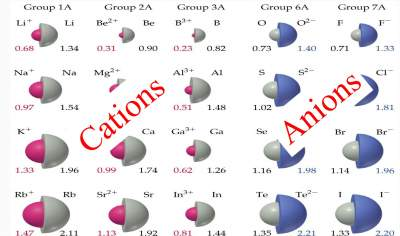Exercise on Cations and Anions

Check your answers here: Solution to the Exercise on Cations and Anions